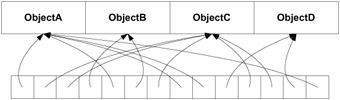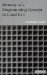# Exercises6.1 Let x and y be two integer variables , and let int* p . Let x = p while y=*p . Are the values of x and y the same? Under what circumstances may they differ ?

6.2 Let int x be an integer array. Let int *p and int *q be pointers. Let p=x and q = &x . Are both pointers pointing to the same location or to different ones?

6.3 Let int *p and let int x = {10,11,12,13} . Let p = &x . What is the value of p ?

6.4 Once I saw the following problem in a C++ code. The programmer had a good idea for a "variable length dynamic array": as long as the array is not too big (10 in our example), store it in a static segment for efficiency; but when it gets too long, use a dynamically allocated segment for the extra storage needed. The class definition is shown here:

` class Array {    public:     Array() { cont = 0; }     ~Array() { if (cont) free(cont); }     char& operator[] (int i) {       if (i < 10)         throw exception;       if (i < 10)         return base[i];       i-=10;       if (cont == 0) {         cont = (char*) malloc(i+1);         length = i+1;         return cont[i];       }       if (length <= i) {         cont = (char*) realloc(cont,(i+1));         length = i+1;         return cont[i];       }       return cont[i];     }     void Strcpy(char* x) { strcpy(base,x); }    protected:      char base;      char *cont;      int length;    };//end class Array `

The program (not shown here) seemed to work fine, but once in a while it behaved erratically, sometimes crashing at the statement a.Strcpy(x); where Array a; and char* x . Can you explain why sometimes it works and sometimes it does not?

6.5 What will be the output of the following program?

` int main()    {      int i, a = {0,1,2};      doit(a,&a);      for(i = 0; i < 3; i++)       printf("a[%d]=%d\n",i,a[i]);      return 0;    }    void doit(int *a, int* b)    {      a = b;      a = b;    } `

6.6 Will this compile? If so, will it run correctly?

` char a = {'('a),'('b),'('c)};    ...    printf("%s\n",a);    ... `

6.7 We have a C program in two separate source files. In the first we define as a global variable a buffer char mybuf; . In the other source file we declare mybuf as external: extern char* mybuf; . When we compile and link our program using a C compiler, the program works correctly, but not when we compile and link it using a C++ compiler. What is your explanation?

6.8 We need a dynamic array of objects, and we expect frequent repetitions. Hence we keep a dynamic array with just one copy of each object and a dynamic array of pointers to the objects in the first array (see Figure 6.5). Because both arrays are supposed to be dynamic when needed, we extend them using realloc() . But sometimes this does not work. Why?Figure 6.5: Dynamic array (Exercise 6.8)Memory as a Programming Concept in C and C++
ISBN: 0521520436
EAN: 2147483647
Year: 2003
Pages: 64

Similar book on Amazon